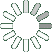Puzzle Command
Daily Puzzle
Number Logic #7
December 20, 2014Find a 6 digit number where:

The 1st digit is the number of 2s in the number.
The 2nd digit is any number.
The 3rd digit is an odd number.
The 4th digit is an odd number.
The 5th digit is equal to 7.
The 6th digit is the number of the 1st and 5th digit.

Each digit is larger than the previous digit.

Puzzle Notes
6 digit numbers range from 100,000 to 999,999.
Enter in your solution below and click submit.